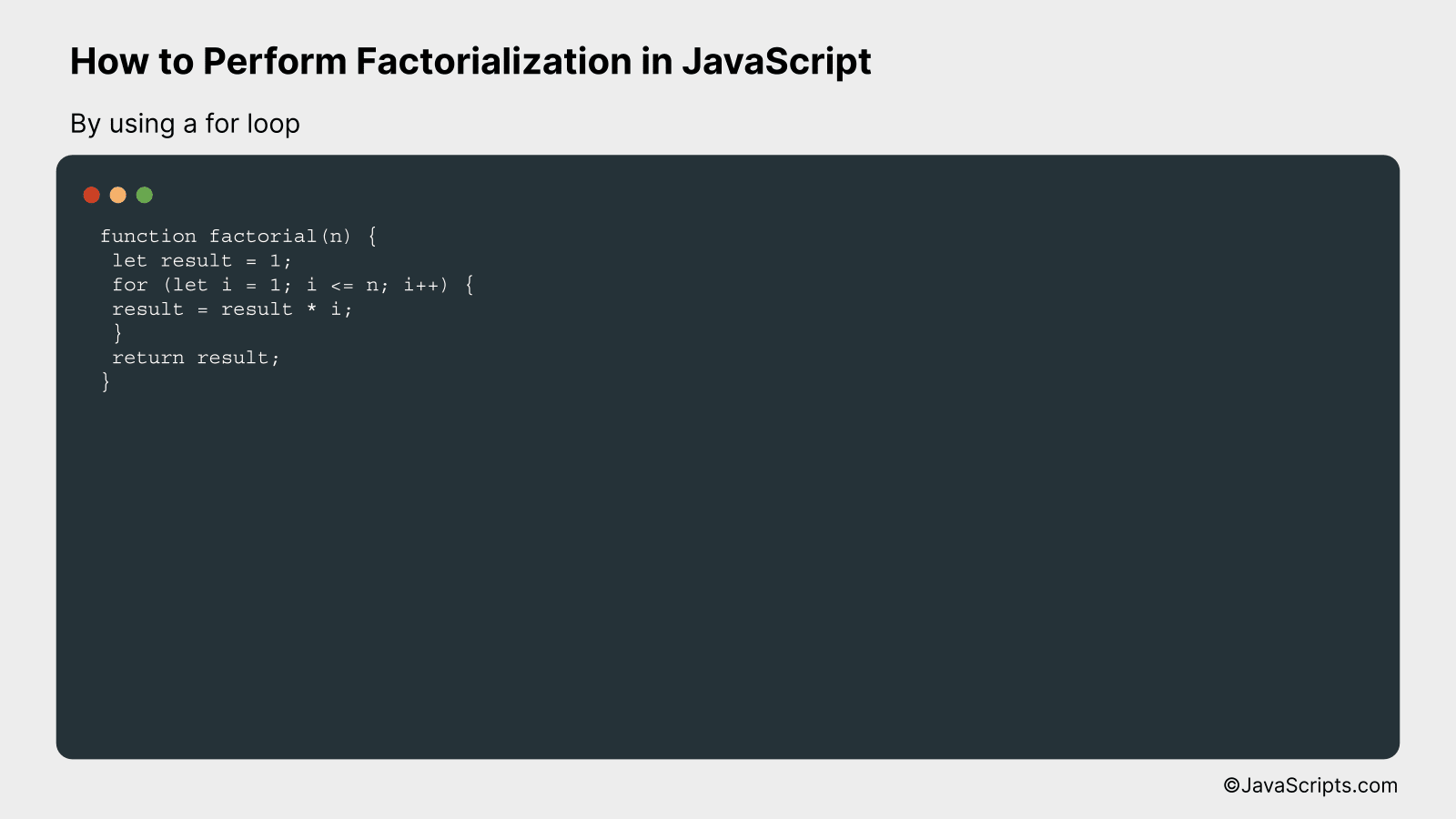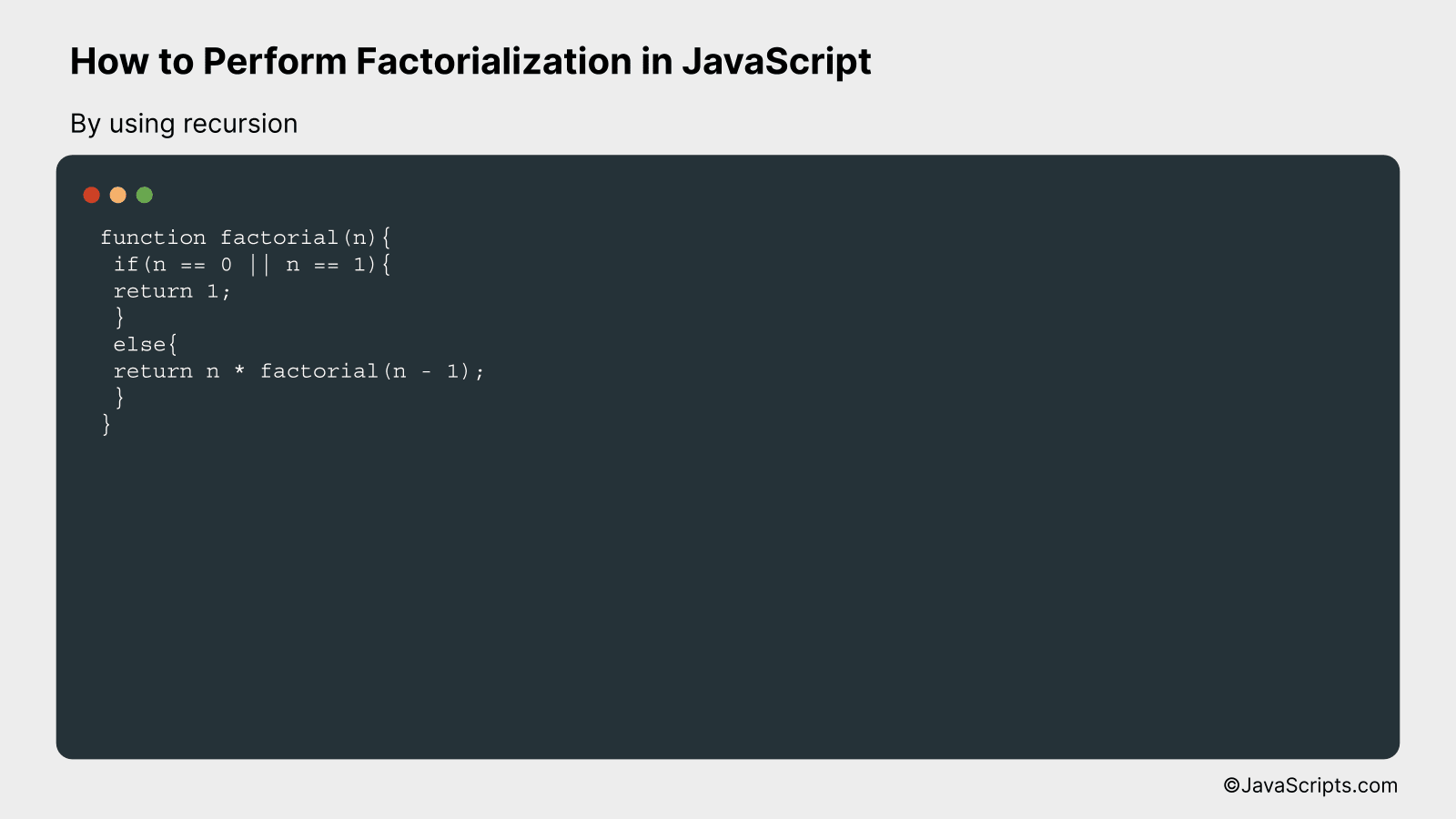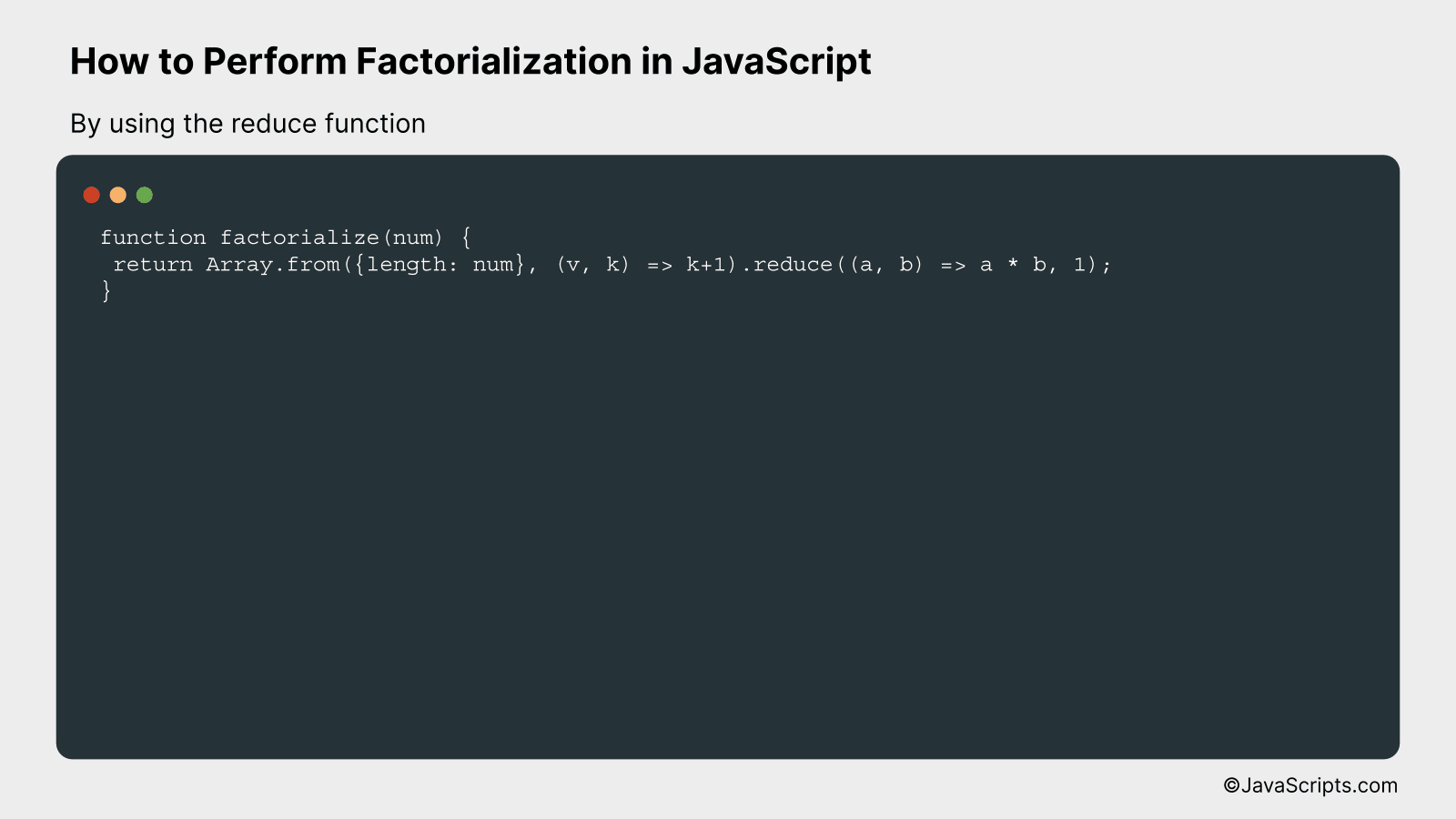# How to Perform Factorialization in JavaScript

Getting to grips with mathematics in programming can sometimes feel like a daunting task, especially if you’re new to the field. Fear not, I’m here to help demystify the concept of factorialization and how it can be performed in JavaScript.

You might remember the factorial operation from your math classes – that exclamation point trailing a number, turning simple digits into a whirlwind of multiplication. By the end of our journey, you’ll be able to comfortably implement this operation into your JavaScript code, even if math isn’t your strong suit.

In this post, we will be looking at the following 3 ways to perform factorialization in JavaScript:

• By using a for loop
• By using recursion
• By using the reduce function

Let’s explore each…

## #1 – By using a for loopThe factorial of a number in JavaScript can be calculated using a ‘for’ loop by initializing a variable to 1 and then multiplying this variable with each number from 1 up to the concerned number. For example, the factorial of 5 (5!) would be 1*2*3*4*5 = 120.

``````
function factorial(n) {
let result = 1;
for (let i = 1; i <= n; i++) {
result = result * i;
}
return result;
}
``````

### How it works

The function 'factorial' takes an input 'n' and calculates the factorial of that number. It uses a 'for' loop to multiply each value from 1 to 'n' together, storing the result in the variable 'result'. After going through all the numbers, it returns the 'result' as the factorial of 'n'.

• Firstly, a function named 'factorial' is declared that takes a number 'n' as input.
• Inside the function, a variable 'result' is initialized to 1. This will hold the final factorial of the number 'n'.
• A 'for' loop is started which runs from 1 to 'n'. The loop counter 'i' is incremented by 1 in each iteration.
• In each iteration of the 'for' loop, 'result' is multiplied by 'i' and the product is stored back in 'result'.
• Once the loop has run 'n' times, which means it has multiplied all numbers from 1 to 'n' together, the final value of 'result' is the factorial of 'n'.
• Finally, the function returns 'result', giving us the factorial of the input number 'n'.

## #2 - By using recursionWe will use the concept of recursion where a function calls itself until it reaches the base case, which in the case of factorialization is when the number reaches 1 or 0.

For example, the factorial of 5 (denoted as 5!) is the product of all positive integers from 1 to 5, which equals 120. This can be represented recursively as follows: 5! = 5 * 4!, and so on until we reach the base case, 1! = 1.

Now, let's look at the code:

``````
function factorial(n){
if(n == 0 || n == 1){
return 1;
}
else{
return n * factorial(n - 1);
}
}
``````

### How it works

In this code, we define a function factorial(n) that computes the factorial of a number n. The function uses recursion, where the function calls itself until it reaches the base case.

Here's a step-by-step explanation:

• The function factorial(n) is defined and takes in an argument n, which is the number we want to find the factorial of.
• A conditional if statement checks if the value of n is 0 or 1, which are our base cases. If n is either 0 or 1, the function returns 1 because the factorial of 0 and 1 is 1.
• If n is neither 0 nor 1, the function returns the product of n and the factorial of n-1. This is the recursive call where the function calls itself.
• The recursion continues until the base case is reached, and the product of all numbers from n down to 1 is returned as the result.
• Thus, the function factorial(n) calculates the factorial of a number n using recursion.

## #3 - By using the reduce functionThe factorial of a number is found by multiplying that number by each number below it until reaching 1. For example, the factorial of 5 (5!) is 5 * 4 * 3 * 2 * 1 = 120. In JavaScript, we can utilize the reduce method to achieve this functionality in a more elegant and functional way.

Here is the JavaScript code to perform factorialization using the reduce function:

``````
function factorialize(num) {
return Array.from({length: num}, (v, k) => k+1).reduce((a, b) => a * b, 1);
}
```
```

### How it works

This function first creates an array with a length of the input number. It then fills this array with the numbers from 1 up to and including the input number. Finally, it uses the reduce function to multiply all of these numbers together.

Here is a step-by-step explanation:

• First, it uses Array.from() to create an array of a specific length. The length of this array is based on the input number.
• Second, it uses a mapping function (v, k) => k+1 to fill this array with numbers from 1 to num. k is the current key/index of the array element that we're processing and v is its value. Since we're interested in filling the array with numbers from 1 to num, we just return k+1.
• Third, it uses reduce() to perform the factorial operation. reduce() takes in a reducer function and an initial value. The reducer function (a, b) => a * b receives two arguments: a is the accumulator that keeps track of the intermediate result, and b is the current element being processed in the array. The initial value is 1 because the multiplication of any number by 1 remains the same.
• Finally, it returns the result of the reduce() operation, which is the factorial of the input number.

Related:

In wrapping up, understanding how to perform factorialization in JavaScript is a valuable skill. It allows you to carry out complex mathematical operations, enhancing your problem-solving capabilities.

Remember, JavaScript is a robust language with immense capabilities. So, keep practicing and exploring its different functionalities to improve your coding abilities.